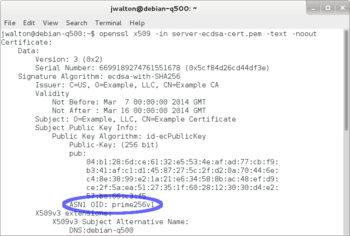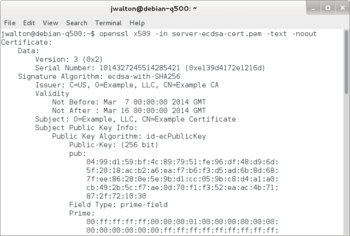# Elliptic Curve Diffie Hellman

Elliptic Curve Diffie Hellman (ECDH) is an Elliptic Curve variant of the standard Diffie Hellman algorithm. See Elliptic Curve Cryptography for an overview of the basic concepts behind Elliptic Curve algorithms.

ECDH is used for the purposes of key agreement. Suppose two people, Alice and Bob, wish to exchange a secret key with each other. Alice will generate a private key dA and a public key QA=dAG (where G is the generator for the curve). Similarly Bob has his private key dB and a public key QB=dBG. If Bob sends his public key to Alice then she can calculate dAQB=dAdBG. Similarly if Alice sends her public key to Bob, then he can calculate dbQA=dAdBG. The shared secret is the x co-ordinate of the calculated point dAdBG. Any eavesdropper would only know QA and QB, and would be unable to calculate the shared secret.

## Using ECDH in OpenSSL

In order for two peers to exchange a shared secret they need to first agree on the parameters to be used. In Elliptic Curve Cryptography this is typically done through the use of named curves. A named curve is simply a well defined and well known set of parameters that define an elliptic curve. OpenSSL has support for a wide variety of different well known named curves. In the example below the ANSI X9.62 Prime 256v1 curve is used.

The example below shows how to set up the parameters based on the use of a named curve, how to generate a public/private key pair for those parameters and subsequently how to derive a shared secret. The details of how to obtain the other party's key (the peer key) are omitted, as this is specific to your particular situation. Note that you do not necessarily need to generate a new private/public key pair for every exchange (although you may choose to do so). Also note that the derived shared secret is not suitable for use directly as a shared key. Typically the shared secret is passed through some hash function first in order to generate a key.

See below for the example code.

```#include <openssl/evp.h>
#include <openssl/ec.h>

unsigned char *ecdh(size_t *secret_len)
{
EVP_PKEY_CTX *pctx, *kctx;
EVP_PKEY_CTX *ctx;
unsigned char *secret;
EVP_PKEY *pkey = NULL, *peerkey, *params = NULL;
/* NB: assumes pkey, peerkey have been already set up */

/* Create the context for parameter generation */
if(NULL == (pctx = EVP_PKEY_CTX_new_id(EVP_PKEY_EC, NULL))) handleErrors();

/* Initialise the parameter generation */
if(1 != EVP_PKEY_paramgen_init(pctx)) handleErrors();

/* We're going to use the ANSI X9.62 Prime 256v1 curve */
if(1 != EVP_PKEY_CTX_set_ec_paramgen_curve_nid(pctx, NID_X9_62_prime256v1)) handleErrors();

/* Create the parameter object params */
if (!EVP_PKEY_paramgen(pctx, &params)) handleErrors();

/* Create the context for the key generation */
if(NULL == (kctx = EVP_PKEY_CTX_new(params, NULL))) handleErrors();

/* Generate the key */
if(1 != EVP_PKEY_keygen_init(kctx)) handleErrors();
if (1 != EVP_PKEY_keygen(kctx, &pkey)) handleErrors();

/* Get the peer's public key, and provide the peer with our public key -
* how this is done will be specific to your circumstances */
peerkey = get_peerkey(pkey);

/* Create the context for the shared secret derivation */
if(NULL == (ctx = EVP_PKEY_CTX_new(pkey, NULL))) handleErrors();

/* Initialise */
if(1 != EVP_PKEY_derive_init(ctx)) handleErrors();

/* Provide the peer public key */
if(1 != EVP_PKEY_derive_set_peer(ctx, peerkey)) handleErrors();

/* Determine buffer length for shared secret */
if(1 != EVP_PKEY_derive(ctx, NULL, secret_len)) handleErrors();

/* Create the buffer */
if(NULL == (secret = OPENSSL_malloc(*secret_len))) handleErrors();

/* Derive the shared secret */
if(1 != (EVP_PKEY_derive(ctx, secret, secret_len))) handleErrors();

EVP_PKEY_CTX_free(ctx);
EVP_PKEY_free(peerkey);
EVP_PKEY_free(pkey);
EVP_PKEY_CTX_free(kctx);
EVP_PKEY_free(params);
EVP_PKEY_CTX_free(pctx);

/* Never use a derived secret directly. Typically it is passed
* through some hash function to produce a key */
return secret;
}
```

You should also refer to the EVP Key Agreement page for general information on the key agreement API in OpenSSL.

## Using the Low Level APIs

Users of the OpenSSL library are expected to normally use the EVP method for working with Elliptic Curve Diffie Hellman as described above and on the EVP Key Agreement page. The EVP API is implemented by a lower level ECDH API. In some circumstances, expert users may need to use the low level API. This is not recommended for most users. However, if you need to use this then an example of use is shown below.

```unsigned char *ecdh_low(size_t *secret_len)
{
EC_KEY *key, *peerkey;
int field_size;
unsigned char *secret;

/* Create an Elliptic Curve Key object and set it up to use the ANSI X9.62 Prime 256v1 curve */
if(NULL == (key = EC_KEY_new_by_curve_name(NID_X9_62_prime256v1))) handleErrors();

/* Generate the private and public key */
if(1 != EC_KEY_generate_key(key)) handleErrors();

/* Get the peer's public key, and provide the peer with our public key -
* how this is done will be specific to your circumstances */
peerkey = get_peerkey_low(key);

/* Calculate the size of the buffer for the shared secret */
field_size = EC_GROUP_get_degree(EC_KEY_get0_group(key));
*secret_len = (field_size+7)/8;

/* Allocate the memory for the shared secret */
if(NULL == (secret = OPENSSL_malloc(*secret_len))) handleErrors();

/* Derive the shared secret */
*secret_len = ECDH_compute_key(secret, *secret_len, EC_KEY_get0_public_key(peerkey),
key, NULL);

/* Clean up */
EC_KEY_free(key);
EC_KEY_free(peerkey);

if(*secret_len <= 0)
{
OPENSSL_free(secret);
return NULL;
}

return secret;
}
```

As noted in the high level EVP section of this page, you should never use a shared secret directly. It must be passed through some form of key derivation function (KDF) first. The last argument to `ECDH_compute_key` can optionally pass a function pointer for such a KDF. The shared secret will then be passed through this function and the value returned in the output buffer will be suitable for direct use as a key.

The function below is taken from `apps/speed.c` in the OpenSSL codebase, and shows an example of a KDF based on the hash function SHA1.

```static const int KDF1_SHA1_len = 20;
static void *KDF1_SHA1(const void *in, size_t inlen, void *out, size_t *outlen)
{
#ifndef OPENSSL_NO_SHA
if (*outlen < SHA_DIGEST_LENGTH)
return NULL;
else
*outlen = SHA_DIGEST_LENGTH;
return SHA1(in, inlen, out);
#else
return NULL;
#endif	/* OPENSSL_NO_SHA */
}
```

SHA1 may not be appropriate if the key length required is longer than the number of bits provided as output from the hash function. A standards based KDF which can be used to derive longer keys is described in: http://www.secg.org/collateral/sec1.pdf (see section 3.6.1)

## ECDH and Named Curves

If you want to save a key and later load it with SSL_CTX_use_PrivateKey_file, then you must set the OPENSSL_EC_NAMED_CURVE flag on the key. You do that by calling EC_KEY_set_asn1_flag(ecKey, OPENSSL_EC_NAMED_CURVE). Failure to do so will result in a SSL error of 0x1408a0c1 (no shared cipher) at the server.

As an example, the following creates a elliptic curve key and saves it using a named curve rather than an expanded list of group paramters:

```EC_KEY *key = NULL;

key = EC_KEY_new_by_curve_name(NID_X9_62_prime256v1);
EC_KEY_set_asn1_flag(key, OPENSSL_EC_NAMED_CURVE);```

If you want to detect the flags after reading a key or certificate from disk, then use the following code:

```int EC_KEY_get_asn1_flag(const EC_KEY* key)
{
if (key)
{
const EC_GROUP* group = EC_KEY_get0_group(key);
if (group)
return EC_GROUP_get_asn1_flag(group);

return 0;
}
}
...

int flags = EC_KEY_get_asn1_flag(ecKey);
ASSERT(flags & OPENSSL_EC_NAMED_CURVE);```

The certificates below were dumped with openssl x509 -in server-ecdsa-cert.pem -text -noout. The certificate on the left was created with a key using OPENSSL_EC_NAMED_CURVE, while the certificate on the right was not. Notice the certificate on the left includes ASN1 OID: prime256v1. The certificate on the left can be used with SSL server using ECDSA, but the certificate on the right cannot because it will result in 0x1408a0c1 at the server.Figure 1: Key with OPENSSL_EC_NAMED_CURVEFigure 2: Key without OPENSSL_EC_NAMED_CURVE

If you use a key or certificate without without the OPENSSL_EC_NAMED_CURVE flag (i.e., one that looks like the image on the right), then the SSL connection will fail with the following symptoms:

```Client (s_client):

```Server (s_server):